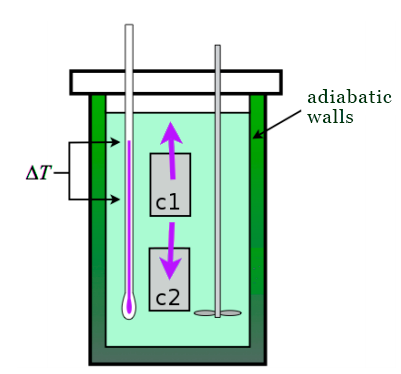Newton's Law of Cooling on a calorimeter with two systems

Having the previous calorimeter with adiabatic walls, we've proven, based on Newton's law of cooling, that the equations are...

$$m_{b} \times C _{pb} \frac{\mathrm{d T_{b}} }{\mathrm{d} t} = k_{t}(T_{c} - T_{b})$$

$$m_{c} \times C _{pc} \frac{\mathrm{d T_{c}} }{\mathrm{d} t} = k_{t}(T_{b} - T_{c})$$

How would it be affected if instead of a single system, it uses two bodies $$c1$$ and $$c2$$ that never get in touch where $$c1$$ has a temperature bigger than the water and $$c2$$ has a temperature that is smaller than the water.$$m_wC_{pw} \frac{dT_w}{dt} = hA\left ( T_{c_1} - T_w \right ) - hA\left ( T_w - T_{c_2} \right )$$
Notice I have introduced the area $A$, since $h\Delta T$ is a flux, or heat transfer per unit area.